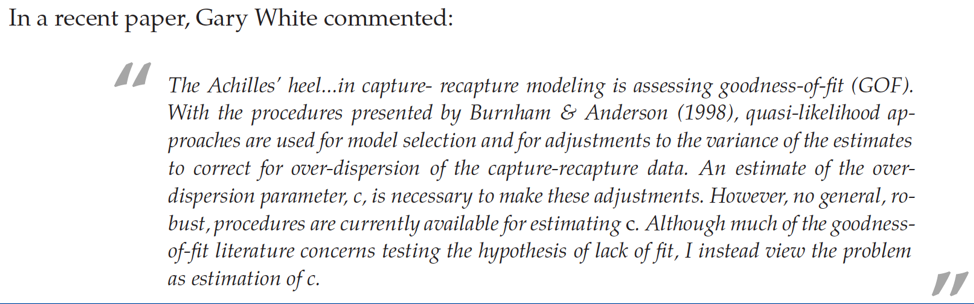Chapter 5 of CW provides information on various approaches for evaluating goodness-of-fit (GOF) for mark-recapture models. Page 1 of that chapter states the following: “… it is a necessary first step to insure that the most general model in your candidate model set (see Chapter 4) adequately fits the data. Comparing the relative fit of a general model with a reduced parameter model provides good inference only if the more general model adequately fits the data.” The most general is your most flexible or complicated model and should fit the best of any model you are evaluating. Other models might be more parsimonious. Unfortunately, GOF testing is not easily done for most mark-recapture model types. We will only scratch the surface of the topic but I do want you to be aware of GOF testing and the ideas behind it.

Pages 5-1 and 5-2 of Chapter 5 of CW, note that, “… by ‘lack of fit’ …we mean that the arrangement of the data do not meet the expectations determined by the assumptions underlying the model.” With respect to the assumptions for live-recaptures models, we are especially concerned that a group of animals being modeled as having the same values for $$\phi_i$$ or $$p_i$$, in fact, is composed of animals that differ with respect to their values for $$\phi_i$$ or $$p_i$$. Specifically, we are concerned that the data are more dispersed (over-dispersed or more variable) than the way they are being modeled. Overdispersion is often termed $$c$$ in the mark-recapture literature and an estimate of the amount of overdispersion is termed $$\hat{c}$$.

On [page 5-6 of CW], the authors provide a noteworthy quote by Gary White (who developed and maintains Program MARK) from an article he published in the Journal of Applied Statistics in 2002 (volume 29, pages 103-106).Although GOF testing is challenging, there are aspects of it that are relatively simple to understand. For example, in linear regression modeling, you encountered the quantity $$R^2$$ where a value of 1.00 is a perfect fit, i.e., all variation in the outcome is explained by the model, i.e., we have a benchmark for a perfect fit. An analog for a CJS model comes from a saturated model, which is one with as many parameters as we have observations. Consider a 3-occasion live-recaptures study for a single group of animals. As you know, you could observe the capture histories 111, 110, 101, 100, 011, and 010. Thus, we could obtain the likelihood value for a model that fits perfectly could be obtained if we could figure out the probabilities ($$prob$$) in the following equation (ignoring the multinomial coefficient).

$\mathcal{L} = prob^{x_{111}}\cdot prob^{x_{110}}\cdot prob^{x_{101}}\cdot prob^{x_{100}}\cdot prob^{x_{011}}\cdot prob^{x_{010}}$

We can do this by substituting the number of individuals with each history ($$Y_\omega$$) over the number released ($$R_i$$) for those probabilities as those reflect a model that fits perfectly. This isn’t a model that can provide us with estimates. Rather, it is a model that provides a benchmark likelihood score. Here’s the log-likelihood version.

$ln\mathcal{L} = Y_{111}\cdot ln \biggl( \frac{Y_{111}}{R_1} \biggr) + Y_{110}\cdot ln \biggl( \frac{Y_{110}}{R_1} \biggr) + Y_{101}\cdot ln \biggl( \frac{Y_{101}}{R_1} \biggr) + Y_{011}\cdot ln \biggl( \frac{Y_{011}}{R_2} \biggr) + Y_{010}\cdot ln \biggl(\frac{Y_{010}}{R_2} \biggr)$

For situations where the $$ln\mathcal{L}$$ for a saturated model can be obtained, you can measure how far the $$ln\mathcal{L}$$ value for a fitted model, e.g., $$\phi_t,p_t$$ is from the $$ln\mathcal{L}$$ for a saturated model. That quantity is called the deviance and you’ll see it in your MARK outputs. Deviance does measure how far a model’s fit is from a perfect fit, but it doesn’t typically provide all that one needs for GOF for most data sets and problems. Much work has focused on estimating overdispersion or $$\hat{c}$$.

Overdispersion can come from lack of independence in the data (e.g., twin deer fawns having similar detection and/or survival outcomes) and unmodeled heterogeneity in rates of detection and/or survival. The effect of overdispersion is to underestimate variances. Take for example, lack of independence: if all of your data come from twins and twins have identical outcomes for detection and survival, once you know one twin’s encounter history, you know the history for the other. Thus, in this extreme (and relatively unlikely) scenario, you only have half as much data as you think you have and your variances, which are estimated based on the number of individuals and not the number of pairs of twins, are too small, which leads to

1. overly precise estimates and

2. over-fitting (choosing models that are overly complex given the actual amount of independent data available). Fortunately, point estimates tend to be unbiased.

If you are in a scenario, where you can come up with $$\hat{c}$$, you can inflate the variances of estimates by $$\hat{c}$$ (and estimated SE’s by $$\sqrt{\hat{c}}$$). Further, you can modify $$AIC_c$$ scores by $$\hat{c}$$ to create $$QAIC_c$$ or quasi-$$AIC_c$$ scores. When $$QAIC_c$$ is used, more complex models are less supported as overdispersion increases.

$QAIC_c = \frac{-2 ln\mathcal{L}}{\hat{c}} + 2k \frac{2k(k+1)}{n-k-1}$

If you are using mark-recapture modeling in your research, I encourage you to read Chapter 5 of CW carefully and to also work with the primary literature that supports the chapter and the topic of GOF for mark-recapture models. You will be learning to use individual covariates in models of $$\phi$$ and $$p$$, which is closely related to GOF. If you have measures for important covariates, you can use them in your models to improve the fit of your models. For example, rather than just modeling $$\phi_{ad-male, yr_i}$$, you can now include information on the animal’s size and other traits. Unfortunately, testing GOF is not simple. But, progress continues to be made on this important topic.